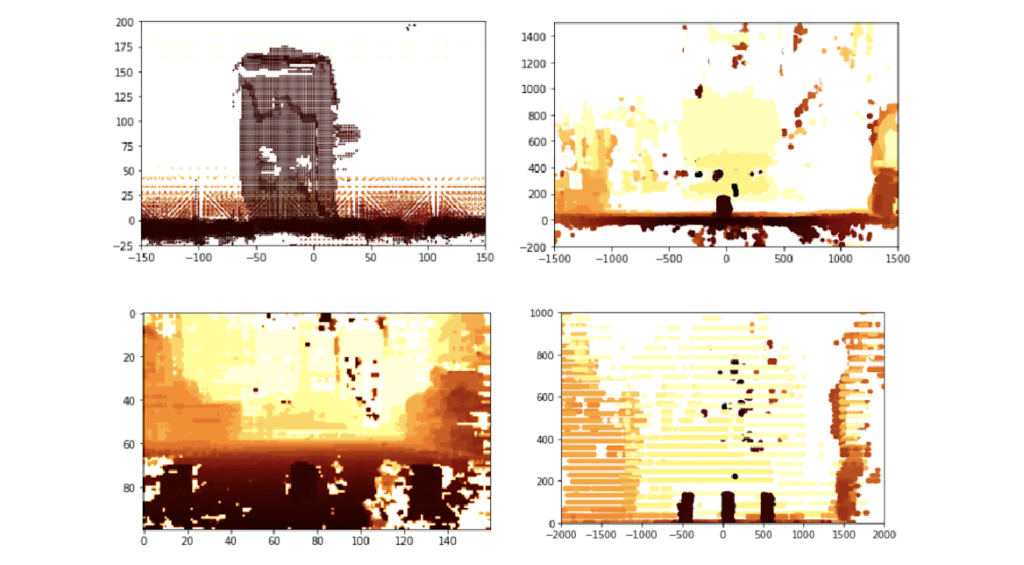+86-13951940532
contact@oakchina.cn

# OAK-D树莓派点云项目

## OAK-D树莓派点云项目“我相信有可能生成非常惊人的点云！”

Richard Hopkins, IBM工程师

`Part 1`

OAK-D是一款超棒的深度相机和AI加速器， 它默认创建的深度流是1280 x 800像素。每个深度测量值都是一个16位无符号整数，代表以毫米为单位的深度（65535表示该像素没有深度信息可用）。在一台功能强大的电脑上，将这些数据与彩色相机结合起来，我相信有可能生成一些非常惊人的点云！

`Part 2`

## 它是如何工作的？

Numpy是一个出色的Python库，它使你能够快速处理多维数组。 这是一种神奇的、强大的、快速的能力——而且它在树莓派上也能工作。

## 代码

1. 前45行只是将OAK-D相机设置为较低分辨率深度模式和每秒10帧。这样做是为了减少数据量，也是为了减少相机可以检测到的最小深度。
2. 第51行抽取输入深度图(抽取变量描述了这种情况发生的程度，从1到20的数字已经过测试，工作正常)
3. 第54到64行完成了将深度图图像中的每个点(被视为2D阵列)转换为三维点(x，y和z)的艰巨工作。
4. 第65至71行将结果点过滤为机器人需要担心的点(机器人两侧超过两米且高于800毫米的点被忽略——这是因为K9高80厘米)
5. 然后，线72至80将x、y、z点排列成一个40×8的10厘米正方形二维网格(400厘米×80厘米)。然后计算网格每一段内所有点的平均距离。
6. 第83行到第87行采用40×8的网格，并进一步简化为一维40位数组；这通过简单地决定40列中的每一列中哪个块是最近的来完成。

``````!/usr/bin/env python3
# coding: utf-8

import cv2
import depthai
import numpy as np
import pandas as pd

device = depthai.Device('', False)
body_cam = device.create_pipeline(config={
"streams": ["depth"],
"ai": {
"blob_file": "/home/pi/depthai/resources/nn/face-detection-retail-0004/face-detection-retail-0004.blob.sh14cmx14NCE1",
"blob_file_config": "/home/pi/depthai/resources/nn/face-detection-retail-0004/face-detection-retail-0004.json",
'shaves' : 14,
'cmx_slices' : 14,
'NN_engines' : 1,
},
"camera": {
"mono": {
# 1280x720, 1280x800, 640x400 (binning enabled)
# reducing resolution decreases min depth as
# relative disparity is decreased
'resolution_h': 400,
'fps': 10
}
}
})

if body_cam is None:
raise RuntimeError("Error initializing body camera")

decimate = 5
max_dist = 4000.0
height = 400.0
width = 640.0
cx = width/decimate/2
cy = height/decimate/2
fx = 1.4 # values found by measuring known sized objects at known distances
fy = 2.05

x_bins = pd.interval_range(start = -2000, end = 2000, periods = 40)
y_bins = pd.interval_range(start= 0, end = 800, periods = 8)

data_packets = body_cam.get_available_data_packets()

for packet in data_packets:
if packet.stream_name == 'depth':
frame = packet.getData()
# Reduce size of depth map
frame = skim.block_reduce(frame,(decimate,decimate),np.min)
height, width = frame.shape
# Convert depth map to point cloud with valid depths
column, row = np.meshgrid(np.arange(width), np.arange(height), sparse=True)
valid = (frame > 200) & (frame < max_dist)
z = np.where(valid, frame, 0)
x = np.where(valid, (z * (column - cx) /cx / fx) + 120 , max_dist)
y = np.where(valid, 325 - (z * (row - cy) / cy / fy) , max_dist)
# Flatten point cloud axes
z2 = z.flatten()
x2 = x.flatten()
y2 = y.flatten()
# Stack the x, y and z co-ordinates into a single 2D array
cloud = np.column_stack((x2,y2,z2))
# Filter the array by x and y co-ordinates
in_scope = (cloud[:,1]<800) & (cloud[:,1] > 0) & (cloud[:,0]<2000) & (cloud[:,0] > -2000)
in_scope = np.repeat(in_scope, 3)
in_scope = in_scope.reshape(-1, 3)
scope = np.where(in_scope, cloud, np.nan)
# Remove invalid rows from array
scope = scope[~np.isnan(scope).any(axis=1)]
# Index each point into 10cm x and y bins (40 x 8)
x_index = pd.cut(scope[:,0], x_bins)
y_index = pd.cut(scope[:,1], y_bins)
# Place the depth values into the corresponding bin
binned_depths = pd.Series(scope[:,2])
# Average the depth measures in each bin
totals = binned_depths.groupby([y_index, x_index]).mean()
# Reshape the bins into a 8 x 40 matrix
totals = totals.values.reshape(8,40)
# Determine the nearest segment for each of the 40
# horizontal segments
closest = np.amin(totals, axis = 0 )
# Round the to the nearest 10cm
closest = np.around(closest,-2)
# Turn into a 1D array
closest = closest.reshape(1,-1)
print(closest)

if cv2.waitKey(1) == ord('q'):
break

del body_cam
del device``````

Tags: